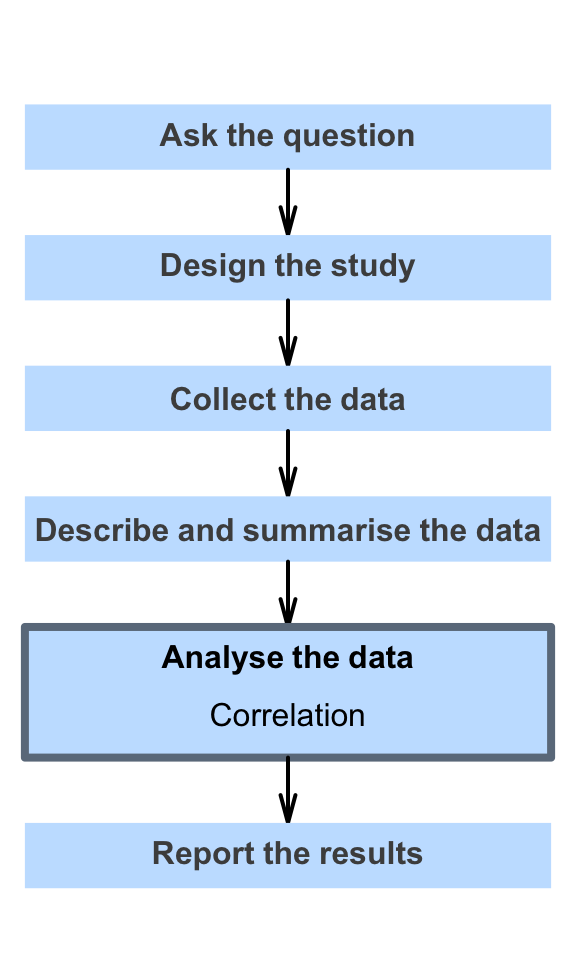# 34 Correlation

So far, you have learnt to ask a RQ, identify different ways of obtaining data, design the study, collect the data describe the data, summarise data graphically and numerically, understand the tools of inference, to form confidence intervals, and to perform hypothesis tests.

In this chapter, you will learn about correlation. You will learn to:

• produce correlation coefficients for exploring the relationship between two quantitative variables.
• produce and interpret $$R^2$$.
• conduct hypothesis tests for correlation coefficients.